# Contextual Parameter Generation for Knowledge Graph Link Prediction

## Basic Idea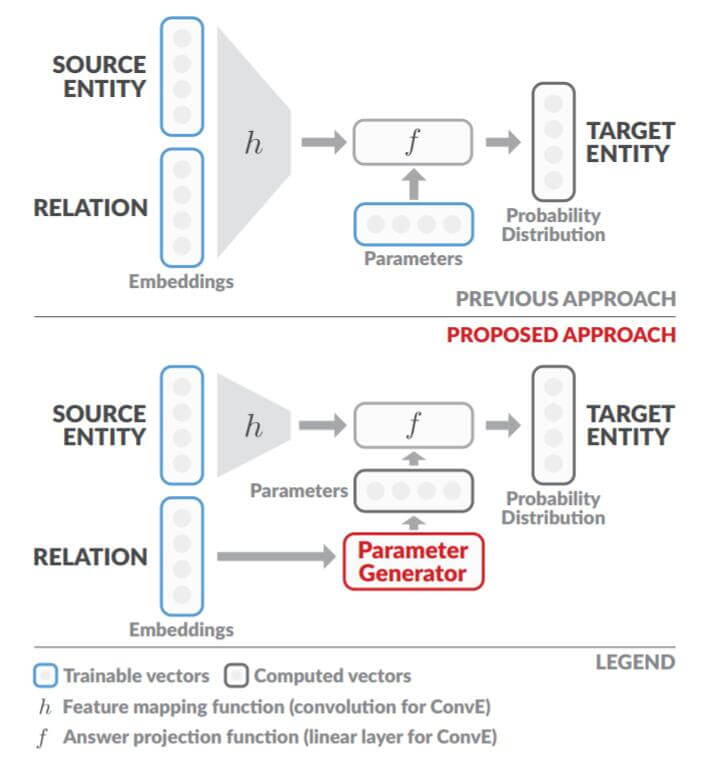## Limited Expressive Power

$$\begin{array}{lr} \mathbf{e}_{s}=\mathbf{E} e_{s}, &\quad \text { (embedding) }\\ \mathbf{r}=\mathbf{R} r, &\quad \text { (embedding) }\\ \mathbf{z}=h_{\phi}\left(\mathbf{e}_{s}, \mathbf{r}, \ldots\right), &\quad\text { (merge) }\\ \text {ans}=f_{\theta}(\mathbf{z}, \ldots), &\quad \text { (prediction) } \end{array}$$

Merge和Prediction的具体操作在不同的KGE方法中通常是不同的, 作者以ConvE为例, 具体的说明了表达是如何被限制的. 在ConvE中, 后两步的数学表达如下:
$$\begin{array}{lr} \mathbf{z}=\operatorname{Conv2D}\left(\operatorname{Reshape}\left(\left[\mathbf{e}_{s} ; \mathbf{r}\right]\right)\right), & \text { (merge) } \\ \hat{e}_{t} =f_{\theta}(\mathbf{z}), & \text { (prediction) } \end{array}$$

ConvE中, Merge Function为将头实体和关系的嵌入表示Concat, 后Reshape成二维数据, 然后用二维卷积获取聚合后的表示$\mathbf{z}$, $f_\theta$ 为一次线性变换, 即$\theta$ 代表投影矩阵.

$$h_{\phi}\left(\mathbf{e}_{s}, \mathbf{r}\right)=\phi \cdot\left[\mathbf{e}_{s} ; \mathbf{r}\right]$$

$[\cdot; \cdot]$ 为Concat, $\phi$ 为单层线性投影. 正是因为Concat在操作上使得$\mathbf{e}_s, \mathbf{r}$ 之间是相互独立的, $h_\phi (\mathbf{e}_s; \mathbf{r})$ 可以被写为$h_\phi (\mathbf{e}_s; \mathbf{r})=\phi_s \mathbf{e}_s + \phi_r\mathbf{r}$, 所以$\mathbf{e}_s, \mathbf{r}$ 只能做加性交互. ConvE在大多数情况下都符合上述情况, 唯独除了卷积核在对$\mathbf{e}_s$ 和$\mathbf{r}$ 的交界处做交互的情况.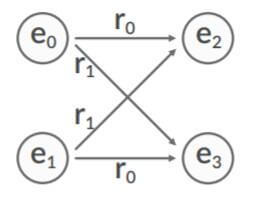$$\begin{array}{l} \mathbf{e}_{2}=\phi_{e} \mathbf{e}_{0}+\phi_{r} \mathbf{r}_{0} \\ \mathbf{e}_{3}=\phi_{e} \mathbf{e}_{0}+\phi_{r} \mathbf{r}_{1} \\ \mathbf{e}_{3}=\phi_{e} \mathbf{e}_{1}+\phi_{r} \mathbf{r}_{0} \\ \mathbf{e}_{2}=\phi_{e} \mathbf{e}_{1}+\phi_{r} \mathbf{r}_{1} \end{array}$$

$$\begin{array}{l} \left(\mathbf{e}_{2}-\mathbf{e}_{3}\right)=\phi_{r}\left(\mathbf{r}_{0}-\mathbf{r}_{1}\right) \\ \left(\mathbf{e}_{3}-\mathbf{e}_{2}\right)=\phi_{r}\left(\mathbf{r}_{0}-\mathbf{r}_{1}\right) \end{array}$$

• $\mathbf{e}_2 = \mathbf{e}_3$: 将导致$\mathbf{e}_2, \mathbf{e}_3$无法区分.
• $\phi_r=0$ 或$\mathbf{r}_0 = \mathbf{r}_1$: 将导致$\mathbf{r}_1, \mathbf{r}_2$ 无法区分或失效.
• $\phi_e=0$ 或$\mathbf{e}_0 = \mathbf{e}_1$: 将导致$\mathbf{e}_0, \mathbf{e}_1$ 无法区分.

## CoPER

### Parameter Generator Network

$$g_{\text {lookup }}(r)=\mathbf{W}_{\text {lookup }} r$$

$$g_{\text {linear }}(r)=\mathbf{W}_{\text {linear }} \mathbf{R} r+\mathbf{b}$$

$$g_{\mathrm{MLP}}(r)=\operatorname{MLP}(\mathbf{R} r)$$

### Enhanced Expressive Power

$$\hat{e}_{t}=f_{\theta}\left(h_{\phi}\left(\mathbf{e}_{s}\right)\right)=f_{\theta}\left(\mathbf{e}_{s}\right)=\theta \mathbf{e}_{s}=(\mathbf{W r}+\mathbf{b}) \mathbf{e}_{\mathbf{s}}$$

$$\begin{array}{l} \mathbf{e}_{2}=\left(\mathbf{W r}_{0}+\mathbf{b}\right) \mathbf{e}_{0} \\ \mathbf{e}_{3}=\left(\mathbf{W r}_{0}+\mathbf{b}\right) \mathbf{e}_{1} \\ \mathbf{e}_{2}=\left(\mathbf{W} \mathbf{r}_{1}+\mathbf{b}\right) \mathbf{e}_{1} \\ \mathbf{e}_{3}=\left(\mathbf{W} \mathbf{r}_{1}+\mathbf{b}\right) \mathbf{e}_{0} \end{array}$$

$$\begin{array}{l} \mathbf{e}_{3}-\mathbf{e}_{2}=\left(\mathbf{W r}_{0}+\mathbf{b}\right)\left(\mathbf{e}_{1}-\mathbf{e}_{0}\right) \\ \mathbf{e}_{3}-\mathbf{e}_{2}=\left(\mathbf{W} \mathbf{r}_{1}+\mathbf{b}\right)\left(\mathbf{e}_{0}-\mathbf{e}_{1}\right) \end{array}$$

$$\mathbf{W}\left(\mathbf{e}_{1}-\mathbf{e}_{0}\right)\left(\mathbf{r}_{0}+\mathbf{r}_{1}\right)+2 \mathbf{b}\left(\mathbf{e}_{1}-\mathbf{e}_{0}\right)=0$$

### CoPER - ConvE

\begin{aligned} \mathbf{z} &=\operatorname{Conv2D}\left(\operatorname{Reshape}\left(\mathbf{e}_{s}\right)\right)\\ \theta &=g(r)\\ \hat{e}_{t} &=f_{\theta}(\mathbf{z})=\theta_{1}+\theta_{2: D_{\theta}} \mathbf{z} \end{aligned}

### CoPER - MINERVA

$$\mathbf{h}_{i}=\mathbf{L S T M}\left(\mathbf{h}_{i-1},\left[\mathbf{e}_{i} ; \mathbf{r}_{i-1}\right]\right)$$

$$\begin{array}{lr} \mathbf{o}_{i}=\operatorname{MLP}\left(\left[\mathbf{h}_{i} ; \mathbf{e}_{i} ; \mathbf{r}_{q}\right]\right), &\quad \text { (merge) }\\ a_{j}=\operatorname{Categorical}\left(\mathbf{A}_{i} \mathbf{o}_{i}\right)&\quad \text { (prediction) } \end{array}$$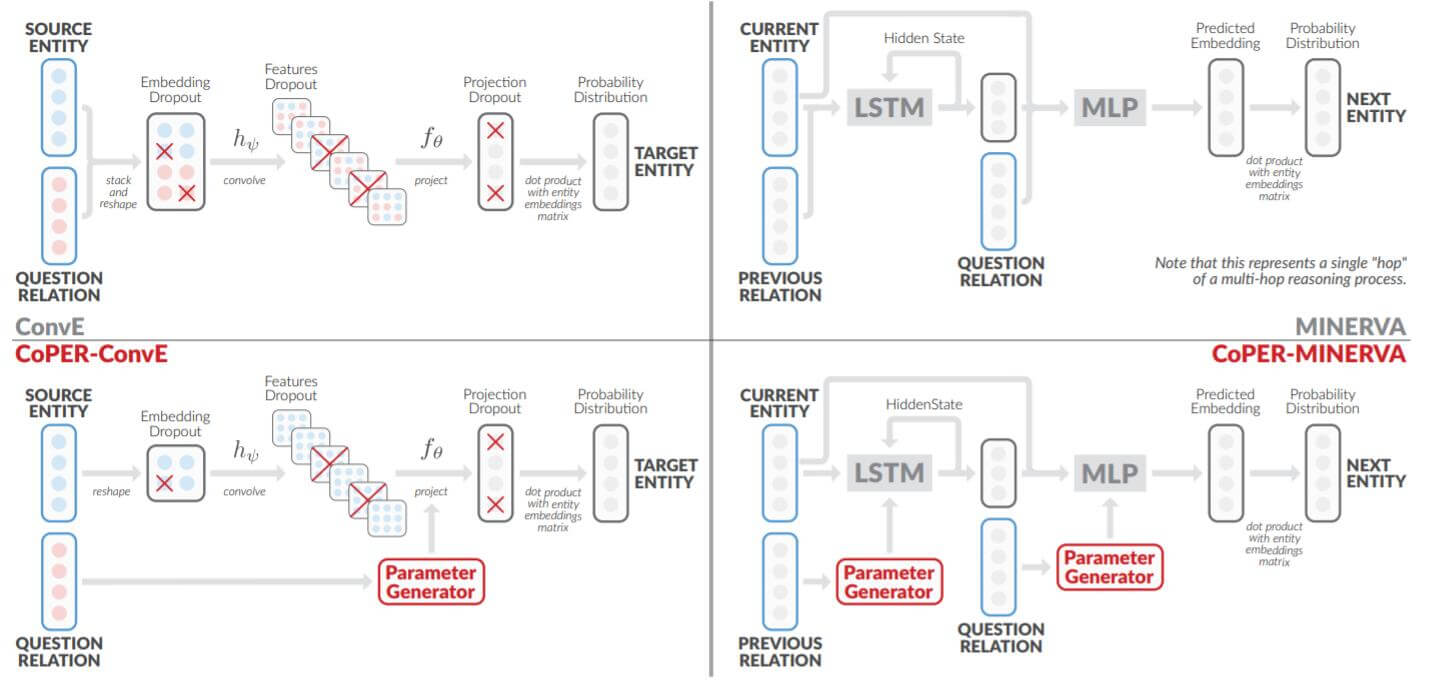## Experiments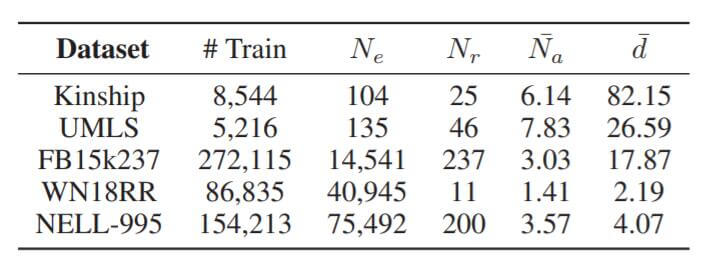$N_e$ 为实体数量, $N_r$ 为关系数量, $\tilde{N}_a$ 为每个问题平均的答案数量, $\tilde{d}$ 为平均节点度.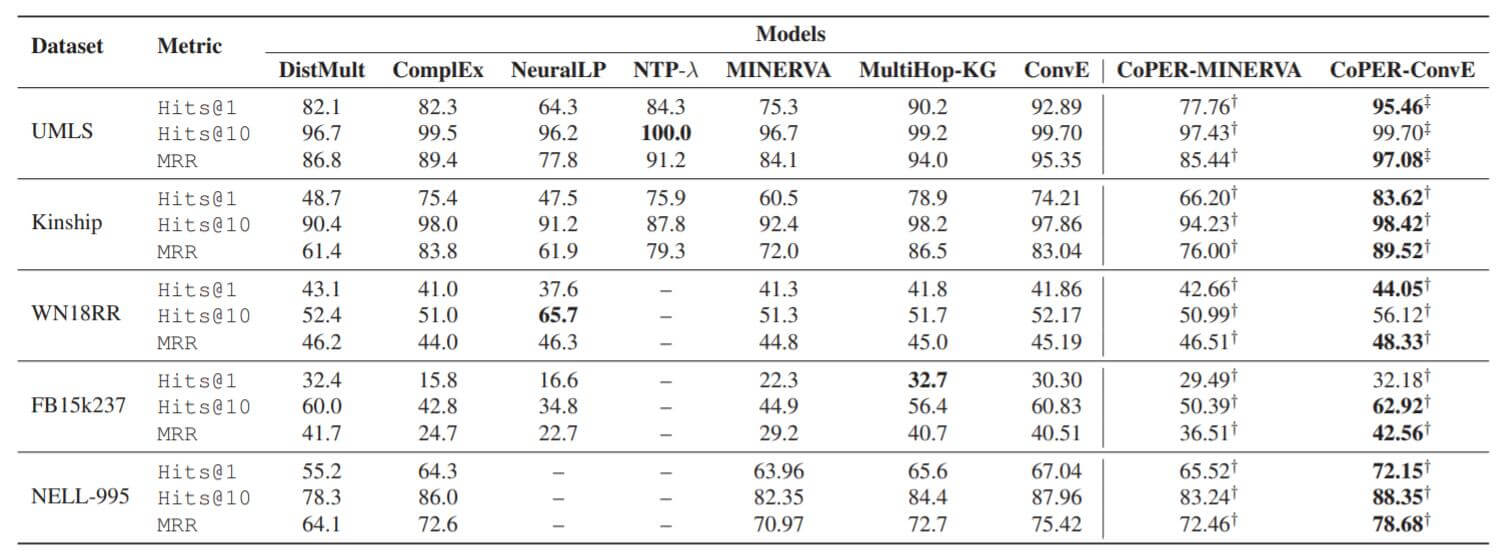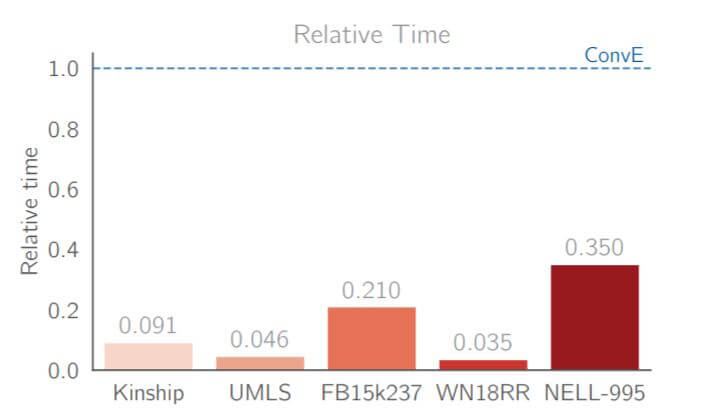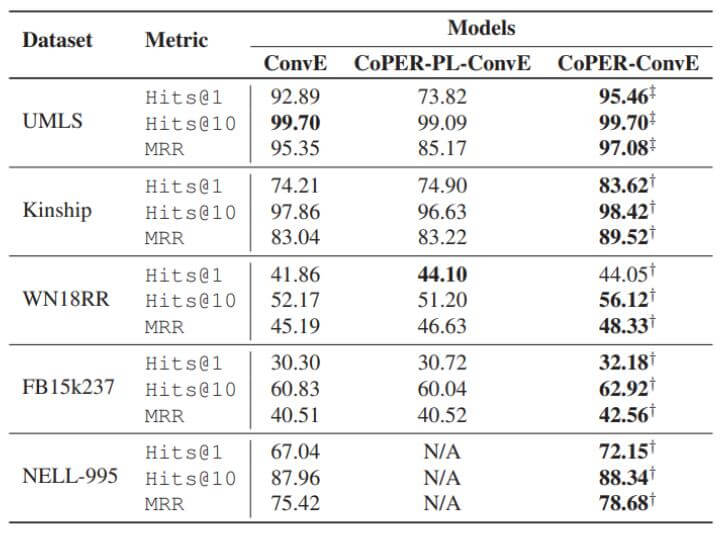“CoPER - PL - ConvE”代表作者前面所述的查表的方法. 而CoPER指的是使用$g_{\text{linear}}$ 或者$g_{\text{MLP}}$ 的方法.

## Summary

CoPER针对作者提出的场景解决方法简单而有效, 将参数生成应用到链接预测任务上, 将关系视为上下文, 生成操作头实体的函数参数.

ConvR侧重于从结构的角度将关系嵌入直接作为卷积核, 而CoPER - ConvE将关系嵌入作为参数生成的上下文依据, 生成投影所需的参数.

上一篇KEQA: Knowledge Graph Embedding Based Question Answering
Knowledge Graph Embedding Based Question Answering本文是论文Knowledge Graph Embedding Based Question Answering的阅读笔记和个人理解. Bas
2021-03-30R-GCN: Modeling Relational Data with Graph Convolutional Networks

2021-03-16
目录